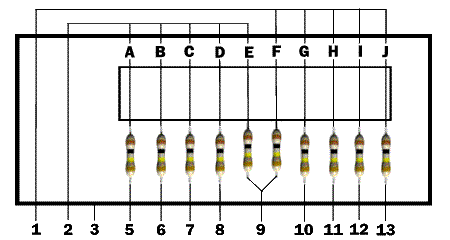E
 FREQ CODE A B C D E F G H I J 67.0 XZ X X X X 71.9 XA X X X X X X X 74.4 WA X X X X X X 77.0 XB X X X X 79.7 WB X X X X X X 82.5 YZ X X X X X 85.4 YA X X X X 88.5 YB X X X X X X 91.5 ZZ X X X X X 94.8 ZA X X X X X X 97.4 ZB X X X X 100.0 1Z X X X X 103.5 1A X X X X X X X 107.2 1B X X X X X X X 110.9 2Z X X X X 114.8 2A X X X X 118.8 2B X X X X 123.0 3Z X X X X 127.3 3A X X X 131.8 3B X X X X 136.5 4Z X X 141.3 4A X X X X X X X X 146.2 4B X X X X X X X 151.4 5Z X X X X X 156.7 5A X X X X X X 162.2 5B X X X X 167.9 6Z X X X X X 173.8 6A X X X X X X 179.9 6B X X X X X X 186.2 7Z X X X X X 192.8 7A X X X X X 203.5 M1 X X X 206.5 8Z X X X X X X 210.7 M2 X X X X X X 218.1 M3 X X X X X X 225.7 M4 X X X X 229.1 9Z X 5 6 7 8 9 10 11 12 13

PROGRAMMING PL

1. Select PL tone required from Frequency chart.

2. Count the number of columns with X's and obtain that number of 100k resistors.

3. Install the resistors as shown in the diagram below in the columns A thru J that have X's from the table.

Example: Tone 229.1 (9Z) requires one 100k resistor between pins 2 and 5. Tone 136.5 (4Z) requires two resistors, one between pins 2 and 9, and one between pins 1 and 10.NOTES
Jumper table for HLN4181A Reedless PL Board

JU1 - Normally Out

JU2 - Normally Out- Install for PL tone disable
JU3 - Normally In - Remove when using external tone
JU4 - Used for "AND" squelch
JU5 - Used for "OR" squelch
JU6 - Normally Out - Install for carrier squelch operations
JU7 - Normally Out - Installed when using external tone
JU8 - Normally In - Remove for reverse burst disable
JU9 - Normally In
"AND" squelch is normally used unless otherwise specified